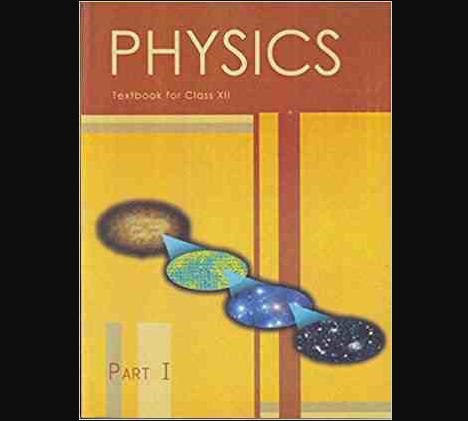# Chapter 7 – Alternating Current Questions and Answers: NCERT Solutions for Class 12 Physics

Class 12 Physics NCERT book solutions for Chapter 7 - Alternating Current Questions and Answers.## 1. A 100Ω100Ω resistor is connected to a 220V220V, 50Hz50Hz ac supply. a) What is the rms value of current in the circuit?

Ans: It is given that,
Resistance, R=100ΩR=100Ω
Voltage, V=220VV=220V
Frequency, f=50Hzf=50Hz
It is known that,
Irms=VrmsRIrms=VrmsR
⇒Irms=220100=2.2A⇒Irms=220100=2.2A
Therefore, the rms value of current in the circuit isIrms=2.2AIrms=2.2A.